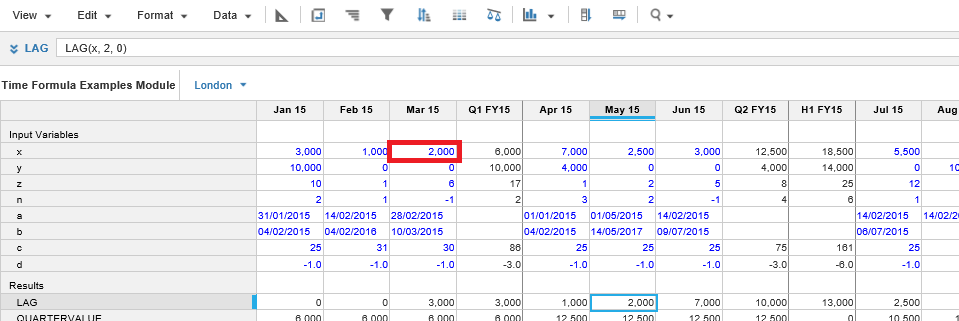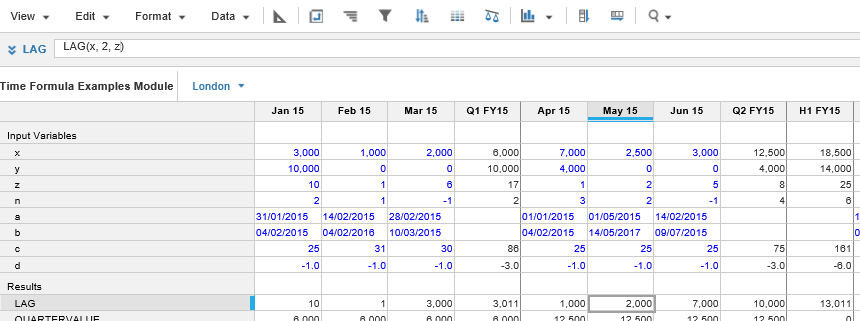1. Calculation functions
2. All Functions
3. Time and Date Functions
4. LAGReturns the source value offset by a specific number of periods. The mode argument controls whether the source can be a non-positive value. Using a positive offset value for LAG refers to earlier periods, a negative value for LAG refers to later periods. A zero value refers to the current period.

The mode argument controls how a non-positive offset value will be treated; In STRICT mode the item to be offset is limited to a line item.

If the offset value is outside the model timescale, the fill value is used instead.

The mode options are NONSTRICT, SEMISTRICT, and STRICT.

• NONSTRICT (default) allows positive, negative and zero values for offset.
• SEMISTRICT ignores negative values for offset and uses the fill value.
• STRICT ignores zero and negative values for offset and uses the fill value.

## Syntax

`LAG(x, n, z,[mode]) `

where:

• x: value: Source line item to be offset
• n: offset: amount of offset
• z: fill: replacement entry if required
• [mode]: NONSTRICT, SEMISTRICT, and STRICT: how the rule is applied

## Format

Input Format Output Format

x: Number - Limited to line item in STRICT mode

n: Number, line item

z: Number

Mode: NONSTRICT, SEMISTRICT, and STRICT

Matches the data format used by argument x

## Arguments

The function uses the following arguments:

• x: Number - Numeric line item
• n: Number - Numeric line item
• z: Number - Numeric line item
• [mode]: Keywords - NONSTRICT, SEMISTRICT, and STRICT

## Constraints

The function has the following constraints:

• The time range providing arguments to a line item must match the time range for the result line item. Any formula that conflicts with this rule will be rejected. For more, see Search.
• A non-time series function, used as a sub-expression of this function, must use the same time range as the line item to which the formula is applied. Combining time series functions and non-time series functions with a time range could return an unexpected result or an error message. For more, see Time Ranges and Time Series Functions.

## Excel equivalent

• No Excel equivalent

## Example

In the example shown below, the formula in the LAG row returns the value from the x row from two periods before the selected cell. If the x value falls outside the range of the current model, the third parameter (0) is used, as seen in Jan 15 and Feb 15 columns. Because mode is not specified, it defaults to NONSTRICT.

Note: The Summary column on the LAG row uses the values for the preceding quarter, it does not apply the LAG formula.
`LAG (x,2,0)   `In this example shown below, the formula in the LAG row returns the value from the x row from two periods before the selected cell. If the x value falls outside the range of the current model, the value from the z column in the current period is used as seen in Jan 15 and Feb 15 columns.

`LAG (x,2,z)`